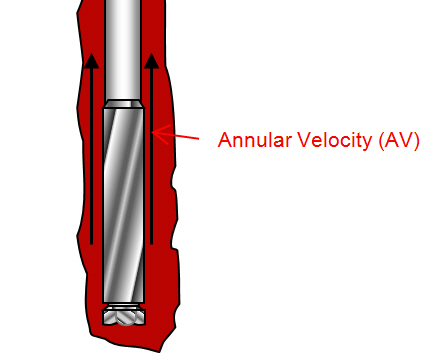## Annular Velocity and Its Importance to Drilling Hydraulics

Annular velocity is the speed of fluid moving up the annulus and it must be high enough to transport cuttings generated while drilling from the wellbore. However, if the annular velocity is too high, it can create hole wash out and excessive equivalent circulating density.

When the drilling mud is circulated through a system, the moving speed is lower at location where the cross section area is bigger. Conversely, when the fluid flows through the small cross section area, the annular velocity at that point is higher.

The cross section area around drill collar and BHA has the smallest area so the annular velocity is the highest.(Annular velocity around drill collar and BHA)

## Annular Velocity (AV) CalculationAnnular Velocity (AV) is how fast of fluid flow in  the annulus while pumping.Three main factors affecting annular velocity are size of hole (bigger ID), size of drill pipe (smaller OD) and pump rate. This post will show you how to calculate annular velocity in feet per minute with different formulas.

# Annular Velocity Formula

## Oilfield Unit

Formula#1: Annular velocity (AV) in ft/min

Annular velocity = Flow Rate÷ Annular Capacity

where;

Annular velocity in ft/min

Flow rate in bbl/min

Annular capacity in bbl/ft

Example:
Flow rate = 10 bbl/min
Annular capacity = 0.13 bbl/ft
AV = 10 bbl/min ÷ 0.13 bbl/ft
AV = 76.92 ft/min

Formula#2: Annular velocity (AV) in ft/min

Annular velocity = (24.5 × Flow Rate) ÷ (Dh2 – Dp2)

where;
Annular velocity in ft/min
Flow Rate in gpm
Dh = inside diameter of casing or hole size in inch
Dp = outside diameter of pipe, tubing or collars in inch

Example:
Flow rate = 800 gpm
Hole size = 10 in.
Drill pipe OD = 5 in.
AV = (24.5 × 800) ÷ (102 – 52)
AV = 261 ft/mim

Formula#3: Annular velocity (AV) in ft/min

Annular Velocity  = Flow Rate × 1029.4÷ (Dh2 – Dp2)

Where;
Annular Velocity in ft/min
Flow Rate in bbl/min

Example:
Flow rate = 13 bbl/min
Hole size = 10 in.
Drill pipe OD = 5 in.
Annular Velocity = 13 bbl/min × 1029.4 ÷ (102 – 52)
Annular Velocity = 178.43 ft/min

## Metric Unit

Formula#1: Annular velocity (AV) in m/min

Annular velocity = Flow Rate÷ Annular Capacity

where;
Annular velocity in m/min
Flow rate in liters/min
Annular capacity in liters/m

Example:
Flow rate = 1600 liters/min
Annular capacity = 68 liters/m
Annular Velocity = 1600 liters/min÷ 68 liters/m
Annular Velocity = 23.5 m/min

Formula#2: Annular velocity (AV) in m/min

Annular velocity = (4000× Flow Rate) ÷ (π×(Dh2 – Dp2))

where;
Annular velocity in m/min
Flow Rate in liters/min
Dh = inside diameter of casing or hole size in mm
Dp = outside diameter of pipe, tubing or collars in mm

Example:
Flow rate = 3000 liters/min
Hole size = 254 mm
Drill pipe OD = 127 mm
Annular Velocity = (4000 × 3000) ÷ (π×(2542 – 1272))
Annular Velocity = 78.9 m/min

Formula#3: Annular velocity (AV) in m/min

Annular Velocity  = (Flow Rate × 4 ×106 ) ÷ (π×(Dh2 – Dp2))

Where;
Annular Velocity in m/min
Flow Rate in m³/min
Dh = inside diameter of casing or hole size in mm
Dp = outside diameter of pipe, tubing or collars in mm

Example:
Flow rate = 2 m³/min
Hole size = 254 mm
Drill pipe OD = 127 mm
AV = (2 m³/min 4 ×106 ) ÷ (π×(102 – 52))
AV = 52.6 m/min

# Flow Rate to Achieve Require Annular Velocity

## Oilfield Unit

Flow rate required in gpm = (Annular Velocity ) × (Dh2 – DP2) ÷ 24.5

Where;
Flow rate in gpm
Annular Velocity in ft/min
Dh = inside diameter of casing or hole size in inch
Dp = outside diameter of pipe, tubing or collars in inch

Example:
Desired annular velocity = 120 ft/mm
Hole size = 10 in
Drill pipe OD = 5 in.
Flow rate required in gpm = 120 × (102– 52) ÷ 24.5
Flow rate required in gpm = 367.4 gpm

## Metric Unit

Flow rate required in gpm = (Annular Velocity × π) × (Dh2 – DP2) ÷ 4000

Where;
Flow rate in liters/min
Annular Velocity in m/min
Dh = inside diameter of casing or hole size in mm
Dp = outside diameter of pipe, tubing or collars in mm

Example:
Desired annular velocity = 37 m/min
Hole size = 254 mm
Drill pipe OD = 127 mm
Flow rate required  = (37 × π) × (2542– 1272) ÷ 4,000
Flow rate required  = 1,406 liters/min

# Stroke per Minute to Achieve Require Annular Velocity

## Oilfield Unit

SPM = (Annular Velocity × Annular Capacity) ÷ Pump Output

Where;
Annular Velocity in ft/min
Annular Capacity in bbl/ft
Pump output in bbl/stk

Example:
Desired annular velocity in ft/min = 120 ft/min
Dh = 12-1/4 in.
Dp = 4-1/2 in.
Annular capacity = 0.1261 bbl/ft
Pump output = 0.136 bbl/stk
SPM = (120 ft/min× 0.1261 bbl/ft) ÷ 0.136 bbl/stk
SPM = 111.3 spm

## Metric Unit

SPM = (Annular Velocity × Annular Capacity) ÷ Pump Output

Where;
Annular Velocity in m/min
Annular Capacity in liters/m
Pump output in liters/stk

Example:
Desired annular velocity in m/min = 46 m/min
Hole size = 254 mm
Drill pipe OD = 127 mm
Annular capacity = 0.0380 m³/m
Pump output = 0.0156 m³/stk
SPM = (46 m/min × 0.0380 bbl/ft) ÷ 0.0156 m³/stk
SPM = 112 spm

Please find the Excel sheet  for calculating annular velocityRef books: Lapeyrouse, N.J., 2002. Formulas and calculations for drilling, production and workover, Boston: Gulf Professional publishing.

Bourgoyne, A.J.T., Chenevert , M.E. & Millheim, K.K., 1986. SPE Textbook Series, Volume 2: Applied Drilling Engineering, Society of Petroleum Engineers.

Mitchell, R.F., Miska, S. & Aadny, B.S., 2011. Fundamentals of drilling engineering, Richardson, TX: Society of Petroleum Engineers.